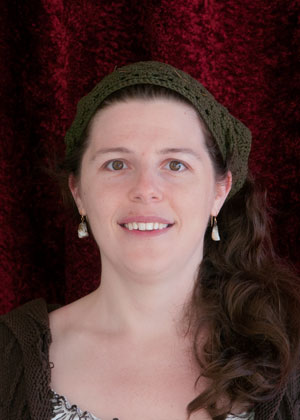# Ruth Vanderpool, Ph.D.

Senior Lecturer
Phone
253-692-4310
Campus Box
358436Degrees
Ph.D.
Mathematics
University of Oregon
2009
M.S.
Mathematics
University of Oregon
2005
B.S.
Mathematics
Pacific Lutheran University
2002
B.A.
Computer Science
Pacific Lutheran University
2002
Introduction

I am an algebraic topologist by training but my research has become more pedagogically focused.

Most recently I have helped develop some of the distance learning curriculum and tracked students' perceptions about mathematics in an interdisciplinary course.

PS: Math is awesome.

Teaching

Classes I have taught:

• Abstract Algebra: Introductory group theory course with a brief introduction to rings and fields.
• Discrete Structures 1: Introductory discrete algebra for computer professionals.
• Linear Algebra: Introductory proof-based course on vector and matrix algebra.
• Calculus 1, 2 and 3:
• Differential calculus and applications for math and science majors.
• Integral calculus and applications for math and science majors.
• Sequences, series and multivariable calculus for science majors.
• Calculus for Life Sciences 2: Integral calculus with an emphasis on applications to the mathematical models used in the life sciences.
• Calculus and Its Practical Applications: Differential calculus with an emphasis on applications to mathematical models used in business & economics.
• Introduction to Statistics: Survey of discrete probability, data analysis, sampling distributions, confidence intervals and hypothesis testing.
• Mathematics for Elementary School Teachers 1, 2 and 3:
• Differential calculus and applications for math and science majors.
• Integral calculus and applications for math and science majors.
• Sequences, series and multivariable calculus for science majors.
• Elementary Functions: Survey of exponential, logarithmic and trigonometric functions.
• College Algebra: Primarily focused on functional formalism and notation.
• Origami Math: Freshmen college orientation course focused on geometry, origami, & scientific thinking.
Affiliations
• Mathematical Association of America (MAA)
• American Mathematical Society (AMS)
• Creative Educational Experiences in Tacoma, WA
Scholarly Interests